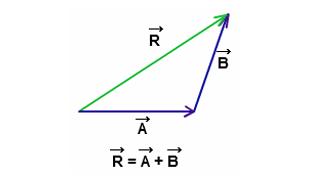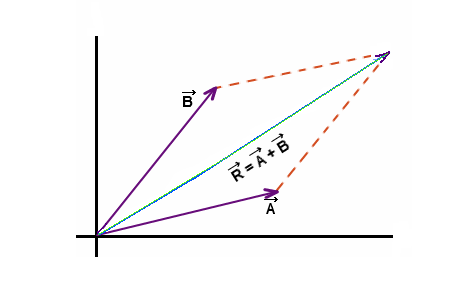# Vector Formulas

A vector is an object that has both a magnitude and a direction. In Geometrically, we can picture a vector as a directed line segment, whose length is the magnitude of the vector and with an arrow indicating the direction.

Vector is typically represented by an arrow whose direction is the same as that of the quantity and whose length is proportional to the quantity magnitude. Vector quantities are often represented by scaled vector diagrams. The vector diagram depicts a displacement vector.

If two forces Vector A and Vector B are acting in the same direction, then its resultant R will be the sum of two vectors.Formula for Triangular law of addition:

$$\begin{array}{l}\vec{R}=\vec{A}+\vec{B}\end{array}$$

If two forces Vector A and Vector B are represented by the adjacent sides of the parallelogram, then their resultant is represented by the diagonal of a parallelogram drawn from the same point.Formula for Parallelogram law of Addition:

$$\begin{array}{l}\vec{R}=\vec{A}+\vec{B}\end{array}$$

### Vector Subtraction

If two forces Vector A and Vector B are acting in the direction opposite to each other then their resultant R is represented by the difference between the two vectors.

Formula for Vector Subtraction:

$$\begin{array}{l}\large \vec{R}=\vec{A}-\vec{B}\end{array}$$

### Solved examples of vector

Example: Give the vector for each of the following:

(a) The vector from (2, -7, 0) to (1, -3, -5)

(b) The vector from (1, -3, -5) to (2, -7, 0)

(c) The position vector for (-90, 4)

Solution:

(a): Remember that to construct this vector we subtract coordinates of the starting point from the ending point.

{1 -2, -3 – (-7), -5 -0} = {-1, 4, -5}

(b): Same thing here: {2 -1, -7 – (-3), 0 (-5)} = {1, -4, 5}

Notice that the only difference between the first two is the signs are all opposite.  This difference is important as it is this difference that tells us that the two vectors point in opposite directions.

(c): Not much to this one other than acknowledging that the position vector of a point is nothing more than a vector with the point’s coordinates as its components. (-90, 4)

 More topics in Vector Formulas Unit Vector Formula Dot Product Formula Cross Product Formula Vector Projection Formula